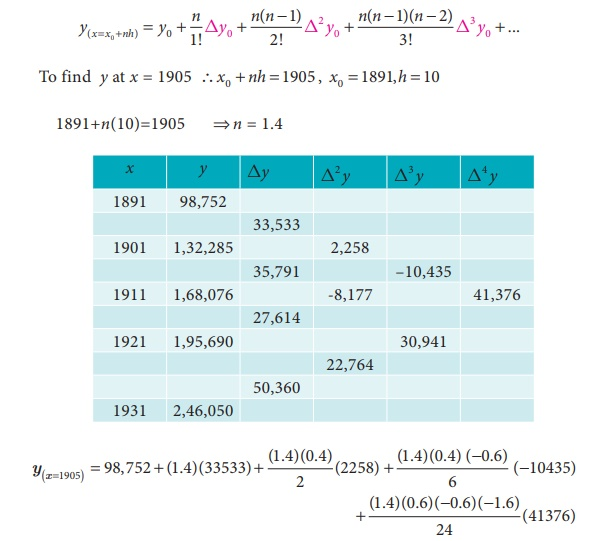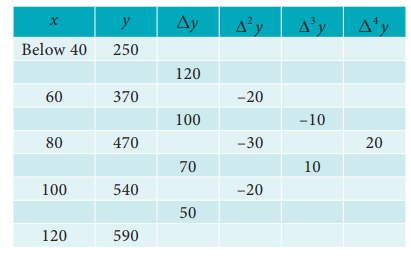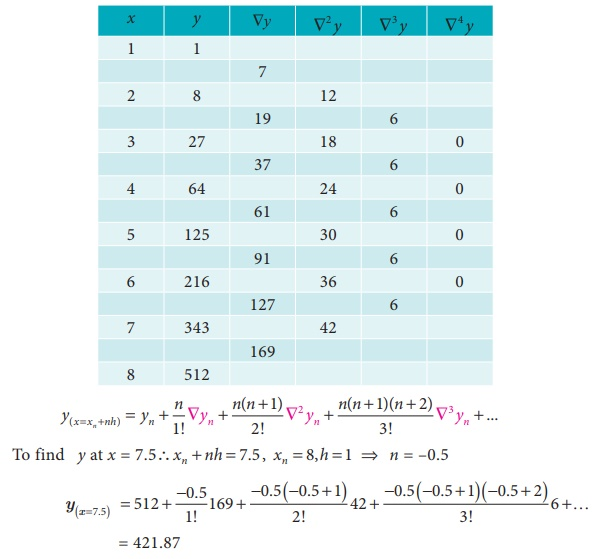Home | | Business Maths 12th Std | Graphical and Algebraic method of Interpolation: Solved Example Problems

# Graphical and Algebraic method of Interpolation: Solved Example Problems

Maths: Numerical Methods: Graphical method, Algebraic method of Interpolation

Example 5.12

Using graphic method, find the value of y when x = 38 from the following data:Solution:

From the graph in Fig. 5.1 we find that for x = 38, the value of y is equal to 35Example 5.13

Using NewtonŌĆÖs formula for interpolation estimate the population for the year 1905 from the table:Solution:

To find the population for the year 1905 (i.e) the value of y at x = 1905

Since the value of y is required near the beginning of the table, we use the NewtonŌĆÖs forward interpolation formula.= 98,752 + 46946.2 + 639.8 + 584.36 + 1390.23

= 1,48,312.59

= 1,48,313

Example 5.14

The values of y = f ( x)for x = 0,1,2, ...,6 are given byEstimate the value of y (3.2) using forward interpolation formula by choosing the four values that will give the best approximation.

Solution:

Since we apply the forward interpolation formula, last four values of f(x) are taken into consideration (Take the values from x = 3).

The forward interpolation formula isExample 5.15

From the following table find the number of students who obtained marks less than 45.Solution:

Let x be the marks and y be the number of students

By converting the given series into cumulative frequency distribution, the difference table is as follows.Example 5.16

Using appropriate interpolation formula find the number of students whose weight is between 60 and 70 from the data given belowSolution:

Let x be the weight and y be the number of students.

Difference table of cumulative frequencies are given below.Let us calculate the number of students whose weight is below 70. For this we use forward difference formula.Number of students whose weight is between

60 and 70 = y(70) ŌłÆ  y(60) = 424 ŌłÆ 370 = 54

Example 5.17

The population of a certain town is as followsUsing appropriate interpolation formula, estimate the population during the period 1946.

Solution:Here we find the population for year1946. (i.e) the value of y at x=1946. Since the value of y is required near the beginning of the table, we use the NewtonŌĆÖs forward interpolation formula.= 20 + 2 - 0.125 + 0.0625 ŌłÆ 0.24609

= 21.69 lakhs

Example 5.18

The following data are taken from the steam table.Find the pressure at temperature t = 1750

Solution:

Since the pressure required is at the end of the table, we apply Backward interpolation  formula. Let temperature be x and the pressure be y.Example 5.19

Calculate the value of y when x = 7.5 from the table given belowSolution:

Since the required value is at the end of the table, apply backward interpolation formula.Example 5.20

From the following table of half- yearly premium for policies maturing at different ages. Estimate the premium for policies maturing at the age of 63.Solution:

Let age = x and premium = y

To find y at x = 63. So apply NewtonŌĆÖs backward interpolation formulaExample 5.21

Find a polynomial of degree two which takes the valuesSolution:

We will use NewtonŌĆÖs backward interpolation formula to find the polynomial.Tags : Numerical Methods , 12th Business Maths and Statistics : Chapter 5 : Numerical Methods
Study Material, Lecturing Notes, Assignment, Reference, Wiki description explanation, brief detail
12th Business Maths and Statistics : Chapter 5 : Numerical Methods : Graphical and Algebraic method of Interpolation: Solved Example Problems | Numerical Methods